# RD Sharma Solutions For Class 7 Maths Chapter 17 Constructions Exercise 17.4

This exercise explains the topic of ASA triangles and steps involved in constructing them. Students can get the detailed RD Sharma Solutions for Class 7 Maths Exercise 17.4 Chapter 17 Constructions from the links available below. RD Sharma Solutions for Class 7 PDF provide the students with a better knowledge about the exercise wise problems covered in this chapter. To score well in the exams, students have to practice the solutions provided by the experts at BYJU’S without fail. PDFs can be downloaded easily from the links given below.

## Download the PDF of RD Sharma Solutions For Class 7 Maths Chapter 17 – Constructions Exercise 17.4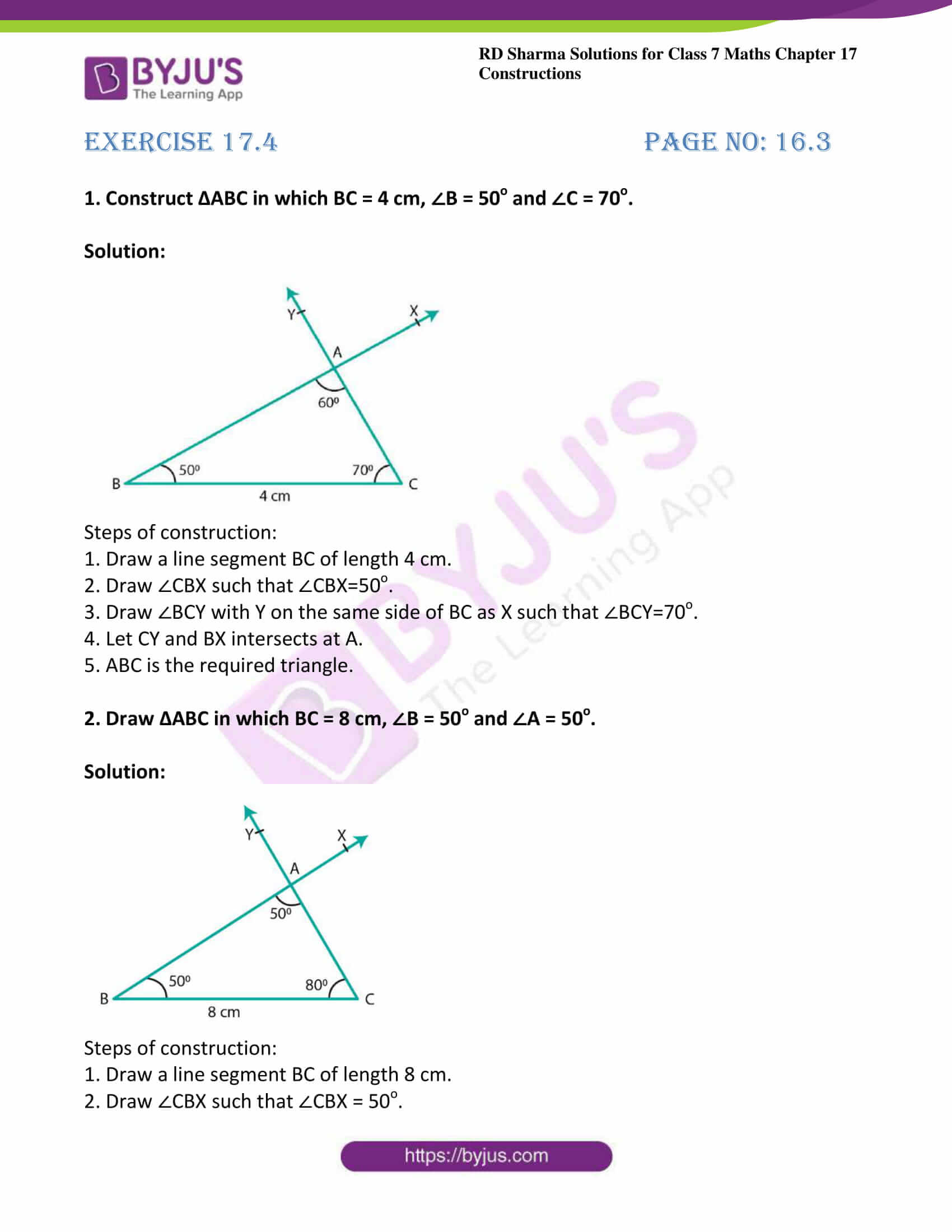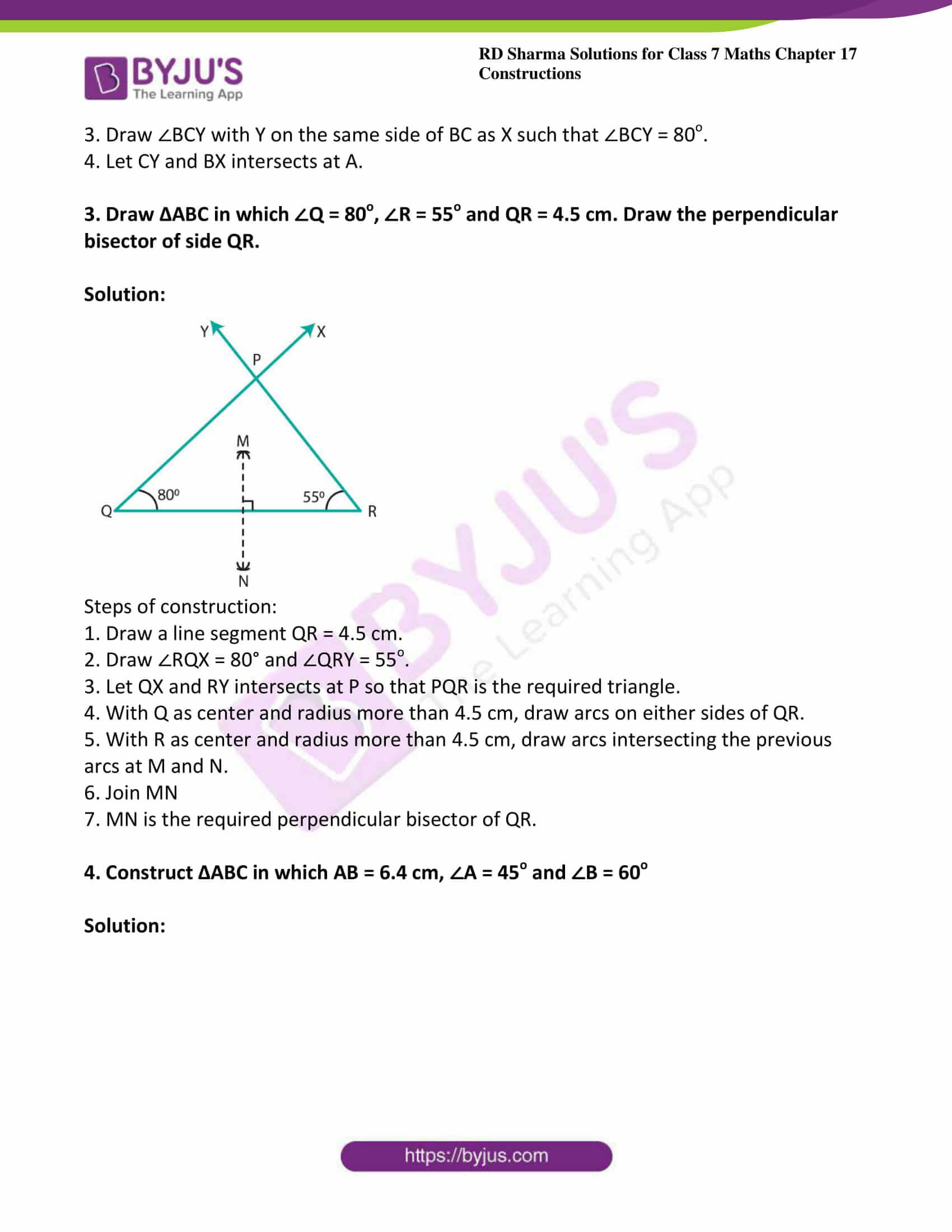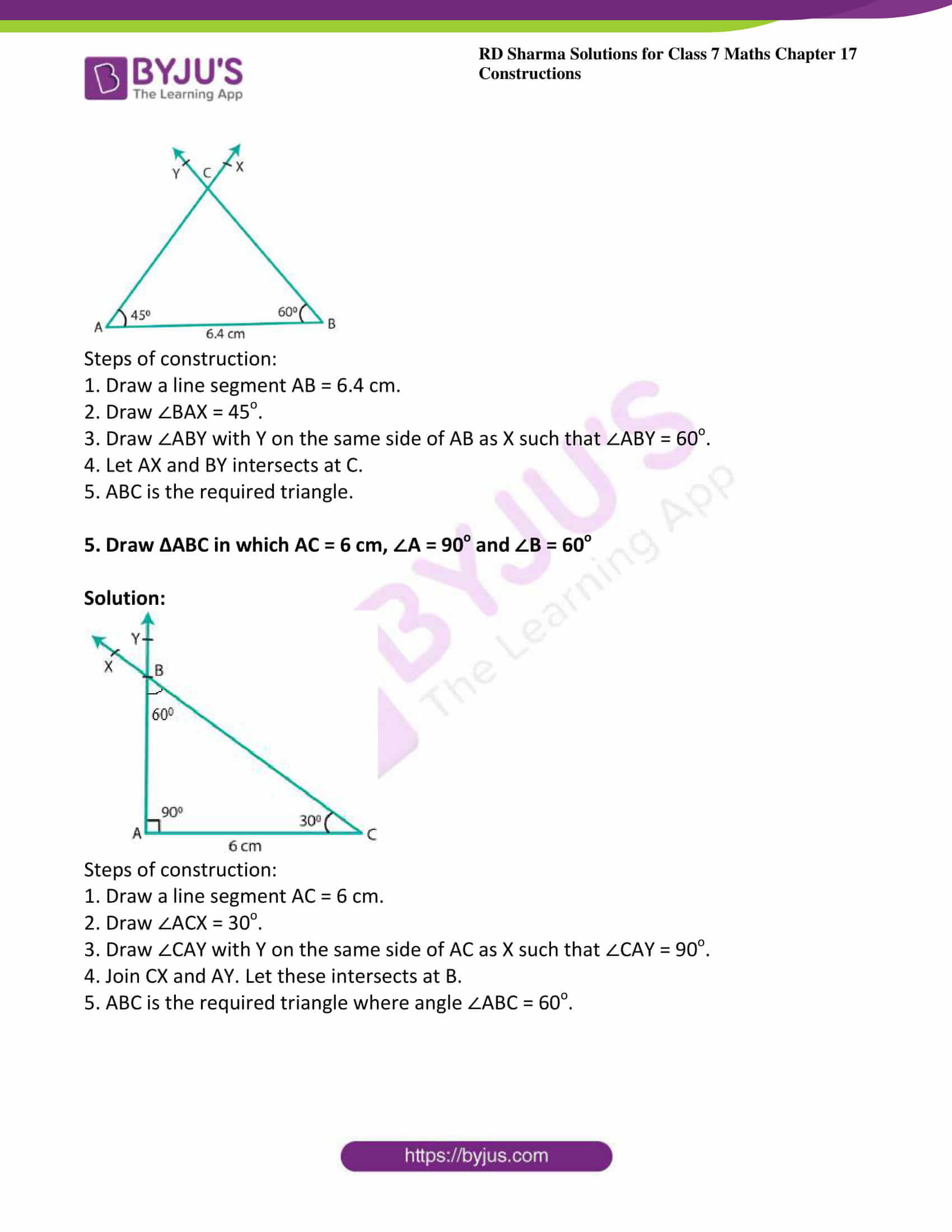### Access answers to Maths RD Sharma Solutions For Class 7 Chapter 17 – Constructions Exercise 17.4

Exercise 17.4 Page No: 16.3

1. Construct ∆ABC in which BC = 4 cm, ∠B = 50o and ∠C = 70o.

Solution: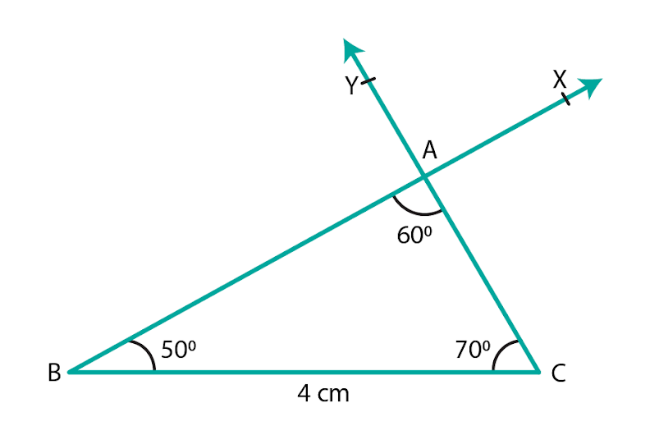Steps of construction:

1. Draw a line segment BC of length 4 cm.

2. Draw ∠CBX such that ∠CBX=50o.

3. Draw ∠BCY with Y on the same side of BC as X such that ∠BCY=70o.

4. Let CY and BX intersects at A.

5. ABC is the required triangle.

2. Draw ∆ABC in which BC = 8 cm, ∠B = 50o and ∠A = 50o.

Solution: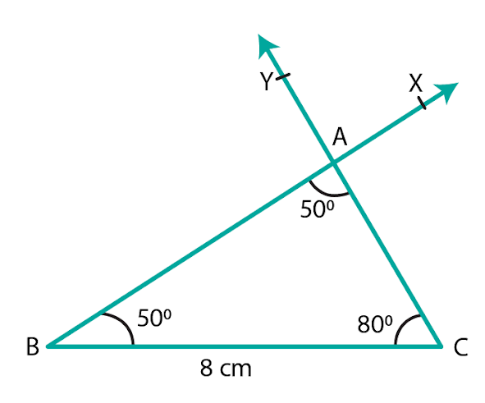Steps of construction:

1. Draw a line segment BC of length 8 cm.

2. Draw ∠CBX such that ∠CBX = 50o.

3. Draw ∠BCY with Y on the same side of BC as X such that ∠BCY = 80o.

4. Let CY and BX intersects at A.

3. Draw ∆ABC in which ∠Q = 80o, ∠R = 55o and QR = 4.5 cm. Draw the perpendicular bisector of side QR.

Solution: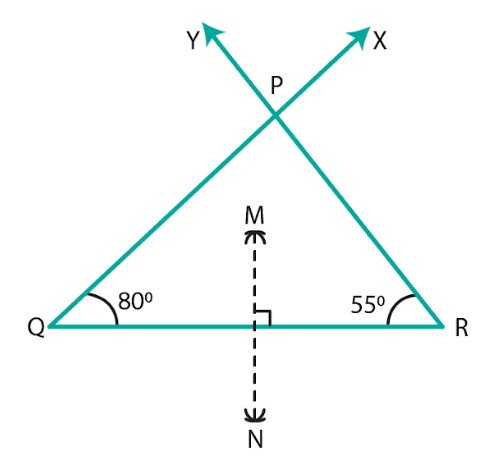Steps of construction:

1. Draw a line segment QR = 4.5 cm.

2. Draw ∠RQX = 80° and ∠QRY = 55o.

3. Let QX and RY intersects at P so that PQR is the required triangle.

4. With Q as center and radius more than 4.5 cm, draw arcs on either sides of QR.

5. With R as center and radius more than 4.5 cm, draw arcs intersecting the previous arcs at M and N.

6. Join MN

7. MN is the required perpendicular bisector of QR.

4. Construct ∆ABC in which AB = 6.4 cm, ∠A = 45o and ∠B = 60o

Solution: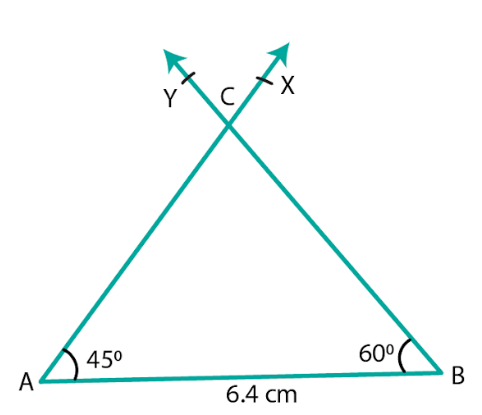Steps of construction:

1. Draw a line segment AB = 6.4 cm.

2. Draw ∠BAX = 45o.

3. Draw ∠ABY with Y on the same side of AB as X such that ∠ABY = 60o.

4. Let AX and BY intersects at C.

5. ABC is the required triangle.

5. Draw ∆ABC in which AC = 6 cm, ∠A = 90o and ∠B = 60o

Solution: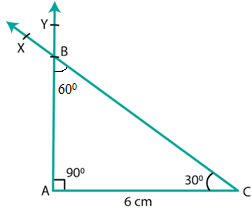Steps of construction:

1. Draw a line segment AC = 6 cm.

2. Draw ∠ACX = 30o.

3. Draw ∠CAY with Y on the same side of AC as X such that ∠CAY = 90o.

4. Join CX and AY. Let these intersects at B.

5. ABC is the required triangle where angle ∠ABC = 60o.